# Compound Interest Calculator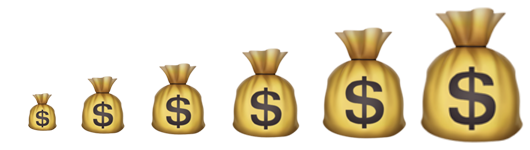Information

## What is compound interest?

A compound interest is interest which calculated not only from initial principal, but also in addition of previous interest.

For example:

My principal is \$10,000 the annual interest rate is 1% and invest it with period of 2 years, the calculation is:

First year: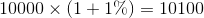Second year: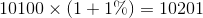Therefore the final amount is \$10,201 and total earnings is \$201

The above calculation can be generalize by: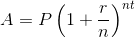where:
A is final amount
P is principal (initial investment)
r is annual nominal interest rate
t is number of years
n is number of compounding periods per year (e.g. it is 12 for monthly compounding)

For compound continuously, the equation is: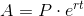where:
A is final amount
P is principal (initial investment)
r is annual nominal interest rate
t is number of years
e is e constant or Euler's number which is roughly equals to 2.718281828459